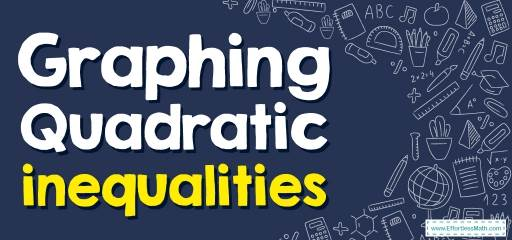# How to Graph Quadratic Inequalities? (+FREE Worksheet!)

Want to know how to graph Quadratic inequalities? You can do it in a few simple and easy steps.## Step by step guide to Graphing Quadratic inequalities

• A quadratic inequality is in the form $$y>ax^2+bx+c$$ (or substitute $$<,≤,$$ or $$≥$$ for $$>$$).
• To graph a quadratic inequality, start by graphing the quadratic parabola. Then fill in the region either inside or outside of it, depending on the inequality.
• Choose a testing point and check the solution section.

### Graphing Quadratic inequalities – Example 1:

Sketch the graph of $$y>3x^2$$.

First, graph $$y=3x^2$$
Since the inequality sing is $$>$$, we need to use dash lines.
Now, choose a testing point inside the parabola. Let’s choose $$(0,2)$$.
$$y>3x^2→2>3(0)^2→2>0$$
This is true. So, inside the parabola is the solution section.

The Absolute Best Book to Ace Pre-Algebra

### Graphing Quadratic inequalities – Example 2:

Sketch the graph of $$y>2x^2$$.

First, graph $$y=2x^2$$
Since the inequality sign is $$>$$, we need to use dash lines.
Now, choose a testing point inside the parabola. Let’s choose $$(0,2)$$. $$y>2x^2→2>2(0)^2→2>0$$
This is true. So, inside the parabola is the solution section.

The Absolute Best Book to Ace the College Algebra Course

## Exercises for Graphing Quadratic inequalities

### Sketch the graph of each function.

• $$\color{blue}{y<-2x^2}$$
• $$\color{blue}{y≥4x^2}$$
• $$\color{blue}{y<-2x^2}$$
• $$\color{blue}{y≥4x^2}$$

The Best Books to Ace Algebra

### What people say about "How to Graph Quadratic Inequalities? (+FREE Worksheet!) - Effortless Math: We Help Students Learn to LOVE Mathematics"?

No one replied yet.

X
45% OFF

Limited time only!

Save Over 45%

SAVE $40 It was$89.99 now it is \$49.99